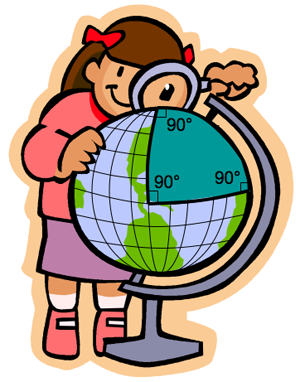## Tutorial 2: Triangles in Curved Space

Curved space is not governed by Euclidean geometry. This means that many of the rules that we take for granted in flat space do not remain true when we allow space to become curved. One example of this is the fact that the three angles in a triangle are no longer required to add up to 180 degrees (in fact, they usually will not).

Imagine starting at the North Pole on a globe and drawing a straight line down to the equator (along one of the longitude lines, or great circles). Now draw another line perpendicular to this line along the equator, reaching through 90 degrees of longitude. Next, draw a line connecting the end of your second line back up to the north pole; you'll notice that your second line is perpendicular to your third line, and that your first and third lines are also perpendicular.Triangle on a Globe
Check that you only used straight lines (defined as lines following great circles around the sphere), and that the three angles between the lines are 90 degrees. If these statements are both true, then you've just drawn a triangle whose angles add up to 270 degrees!

You can observe the same phenomenon in the applet. First, turn the trail for the turtle on by clicking the "trail on" button. Next, move the turtle forward 90 units (this moves the turtle through 90 degrees along the surface of the sphere) either by setting the step size to 90 units and taking 1 step forward or by setting the step size to something smaller (default is 10 units) and taking several steps forward (9 steps for a step size of 10 units). After you have moved forward by 90 units, rotate 90 degrees by setting the rotating angle to 90 degrees (this is the default) and pressing the rotate button. After you have finished rotating, move forward 90 units a second time, rotate by 90 again, and then move forward by 90 units a third time. Check to see that the last angle in the triangle is 90 degrees by rotating 90 degrees and seeing if the first side is straight in front of you again. If you are not sure that all the lines are straight, walk through the triangle again to convince yourself that you were indeed walking straight along each side. (Notice that the interior angle between each pair of lines is actually 180 degrees minus the angle you rotated through. Since you rotated by 90 degrees each time, the interior angle of the triangle was also 90 degrees. For any other triangle however, the rotation angle and interior angle will be different; for example, to achieve an interior angle of 50 degrees, a rotation of 130 degrees must be performed.)

You just created 3 straight lines with angles of 90 degrees between them, yet a triangle was created instead of the open-topped box you would expect in flat space! This means that the triangle we created had angles that summed to 270 degrees instead of 180 degrees. You could imagine that, depending on how big the triangle's sides were, you could create a triangle whose angles summed to almost any number; check to see that this is true by creating triangles of your own. One easy way to make different triangles is to use the following procedure:

1. Move 90 units
2. Rotate 90 degrees
3. Move X units (any value you want, but values less than 180 will be easiest to see)
4. Rotate 90 degrees
5. Move 90 units
The measure of the angle opposite the side with length X will be X degrees. Why is this true? Can you come up with other methods for creating triangles? Are there any limits on the sum of the angles of triangles in the geometry presented in this applet?

Copyright (C) 2010 Stephanie Erickson, Gary Felder
Terms and Conditions of Use